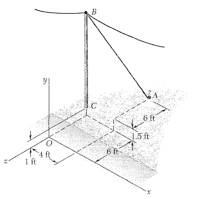# Moment of a pole of unknown height

PensNAS

## Homework Statement

Utility Pole BC is guyed by cable AB as shown. The tension in cable AB (TAB) acting at point B creates a moment about point O (MO) equal to -900i+Myj-315k lb-ft, where My is unknown.
a) What is the length of the pole (LBC)?
b) What is the magnitude of the tension, TAB?MOABxF
TAB=uAB|TAB|

## The Attempt at a Solution

Vector BO <0,-(h+1),6>
Vector BA <4,-h,-6>
|BA|= $\sqrt{h^{2}+52}$
TBA= TBA$\frac{<4,-h,-6>}{|BA|}$

I cross multiplied and got TBA<$\frac{12h+6}{|BA|}$,$\frac{24}{|BA|}$,$\frac{4h+4}{|BA|}$>

In order to solve for the height of the pole, I need TBA. Where i get stuck is here, and a lack of confidence that my vectors BA and BO are correct. In order to solve for the height of the pole, I need the tension, but that is the second part of the problem.

EDIT: tips on how to fix the itex tags would be appreciated,too.

Last edited:

Homework Helper
Gold Member

## The Attempt at a Solution

Vector BO <0,-(h+1),6>
Do you need vector BO or vector OB?

Vector BA <4,-h,-6>
Check the diagram carefully to see if the y-displacement from B to A is -h.

In order to solve for the height of the pole, I need the tension, but that is the second part of the problem.
You will have to solve 2 equations simultaneously for h and TAB.

EDIT: tips on how to fix the itex tags would be appreciated,too.
When writing latex, don't use the superscript and subscript buttons on the tool bar. Instead, use standard latex notation: h^2, for example.

•1 person
PensNAS
Vector OB should be the one I need, which is <0,h+1,-6> Vector BA should be <4,0.5-h,-6>.

This gives a new cross product of T$_{BA}$<$\frac{-12h-3}{|BA|}$,$\frac{-24}{|BA|}$,-$\frac{4h-4}{|BA|}$>

Am I right in thinking I can then set the i and k components of M$_{O}$ equal to the i and k components of the cross product? If so then I get:

From the i component:
T$^{2}_{BA}$:$\frac{90000(h^{2}-h+52.25)}{16h^{2}+h+52.25}$

From the k component:
T$^{2}_{BA}$:$\frac{6202(h^{2}-h+52.25)}{h^{2}+2h+1}$

Do I set those equal to each other? If so, I get h=14.74 feet. Plugging that value for h into
-900=T$_{BA}$$\frac{-12h-3}{|BA|}$ or
-315=T$_{BA}$$\frac{-4h-4}{|BA|}$
gives me T$_{BA}$=84.6 lb

Last edited:
Homework Helper
Gold Member
Vector OB should be the one I need, which is <0,h+1,-6> Vector BA should be <4,0.5-h,-6>.

This gives a new cross product of T$_{BA}$<$\frac{-12h-3}{|BA|}$,$\frac{-24}{|BA|}$,-$\frac{4h-4}{|BA|}$>

Are you sure about the negative sign in the numerator of the last term? Shouldn't it be 4h + 4 instead of 4h - 4?

Am I right in thinking I can then set the i and k components of M$_{O}$ equal to the i and k components of the cross product? If so then I get:

From the i component:
T$^{2}_{BA}$:$\frac{90000(h^{2}-h+52.25)}{16h^{2}+h+52.25}$

I don't agree with your denominator here.

From the k component:
T$^{2}_{BA}$:$\frac{6202(h^{2}-h+52.25)}{h^{2}+2h+1}$

Do I set those equal to each other? If so, I get h=14.74 feet.

I agree with your value of h ( I get 14.75 ft ).

Plugging that value for h into
-900=T$_{BA}$$\frac{-12h-3}{|BA|}$ or
-315=T$_{BA}$$\frac{-4h-4}{|BA|}$
gives me T$_{BA}$=84.6 lb

I get somewhat less than 84.6 lb. But your approach seems correct.
[EDIT: Note that if you take the ratio of your last two equations, you get a simple equation for finding h.]

•1 person
PensNAS
4h+4 is correct, I dropped a negative.

The denominator was a typo. I have it on my paper as $\frac{90000(h^{2}-h+52.25)}{16h^{2}+8h+1}$.

I redid the calculation for the tension and got 79.5 lb.
It would seem I got sloppy, this was the 8th time working through the problem.Thanks for the help!

Homework Helper
Gold Member
OK. I got 79.9 lb. Good work.

Pensacola?

PensNAS
I get that when I spend the extra 15 seconds to calculate |BA| further than 15.9.

Pensacola?

Yep, home to beaches and Naval Aviation.